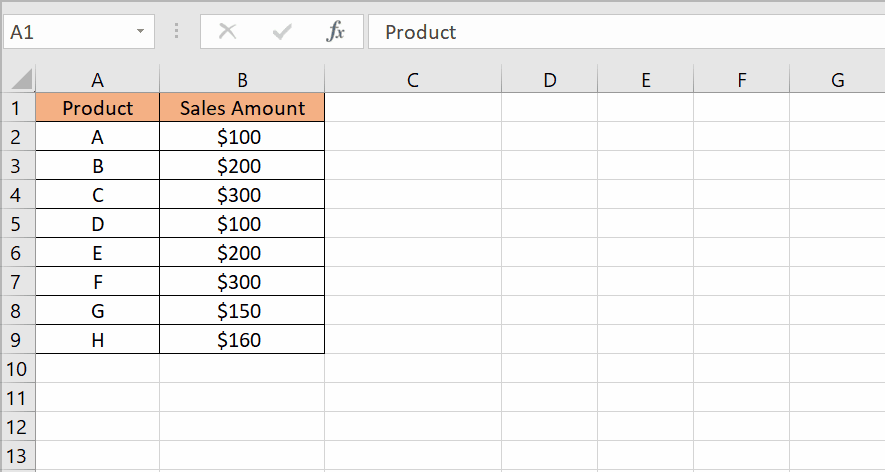# How to sum unique values in Excel.

Suppose you have an Excel sheet containing the following column: Product, and Sales Amount. In this tutorial, we will explore various methods to sum unique values in Excel and demonstrate their application using a diverse dataset. Now let’s take a look at the Dataset.When working with data in Excel, there are situations where you may need to sum only the unique values in a specific range or column. Summing unique values allows you to aggregate data without duplication, providing more accurate insights and streamlined data analysis.

### Step – 1 Insert the formula.– Select the cell where you want to write the formula.
– The syntax of this formula will be
=SUMPRODUCT((COUNTIF(Range,Range)=1)*Range)
– In our case, the formula will be
=SUMPRODUCT((COUNTIF(B2:B9,B2:B9)=1)*B2:B9)

### Step – 2 Apply the formula.– Press Enter to apply the formula.
– The unique values will be added.